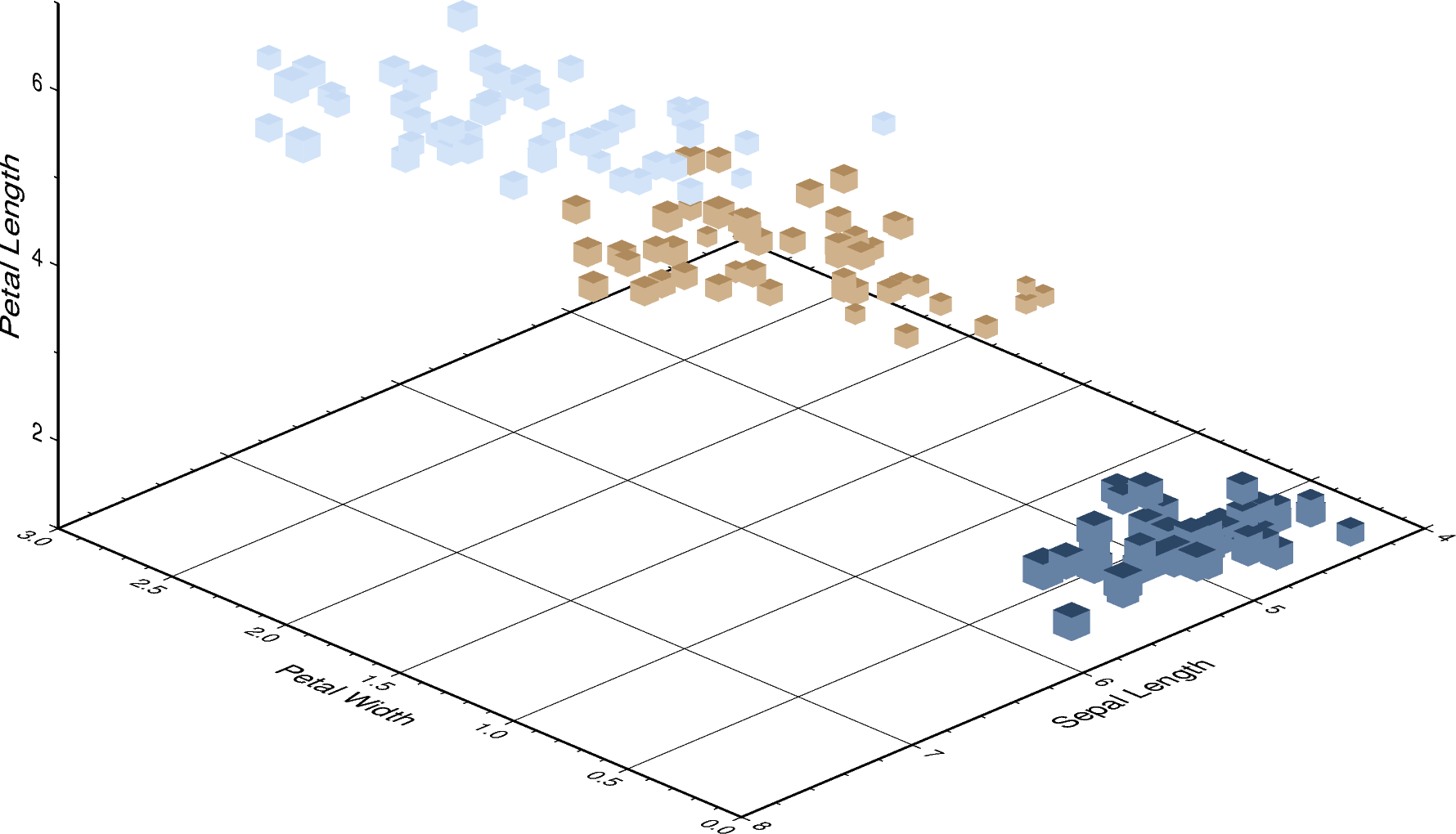# 3D Scatter plots¶

The pygmt.Figure.plot3d method can be used to plot symbols in 3D. In the example below, we show how the Iris flower dataset can be visualized using a perspective 3-dimensional plot. The region argument has to include the $$x$$, $$y$$, $$z$$ axis limits in the form of (xmin, xmax, ymin, ymax, zmin, zmax), which can be done automatically using pygmt.info. To include the z-axis stick, set frame as a minimum to something like frame=["WsNeZ", "zaf"]. Use perspective to control the azimuth and elevation angle of the view, and zscale to adjust the vertical exaggeration factor.Out:

<IPython.core.display.Image object>


import pandas as pd
import pygmt

# Load sample iris data, and convert 'species' column to categorical dtype
df["species"] = df.species.astype(dtype="category")

# Use pygmt.info to get region bounds (xmin, xmax, ymin, ymax, zmin, zmax)
# The below example will return a numpy array like [0., 3., 4., 8., 1., 7.]
region = pygmt.info(
table=df[["petal_width", "sepal_length", "petal_length"]],  # x, y, z columns
per_column=True,  # report output as a numpy array
spacing="1/2/0.5",  # rounds x, y and z intervals by 1, 2 and 0.5 respectively
)

# Make our 3D scatter plot, coloring each of the 3 species differently
fig = pygmt.Figure()
pygmt.makecpt(cmap="cubhelix", color_model="+c", series=(0, 3, 1))
fig.plot3d(
x=df.petal_width,
y=df.sepal_length,
z=df.petal_length,
sizes=0.1 * df.sepal_width,  # Vary each symbol size according to a data column
color=df.species.cat.codes.astype(int),  # Points colored by categorical number code
cmap=True,  # Use colormap created by makecpt
region=region,  # (xmin, xmax, ymin, ymax, zmin, zmax)
frame=[
"WsNeZ3",  # z axis label positioned on 3rd corner
'xafg+l"Petal Width"',
'yafg+l"Sepal Length"',
'zafg+l"Petal Length"',
],
style="uc",  # 3D cUbe, with size in centimeter units
perspective=[315, 25],  # Azimuth NorthWest (315°), at elevation 25°
zscale=1.5,  # Vertical exaggeration factor
)
fig.show()


Total running time of the script: ( 0 minutes 0.875 seconds)

Gallery generated by Sphinx-Gallery